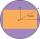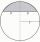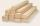# Pythagorean theorem - math word problems

1. Concentric circlesIn the circle with diameter 19 cm is constructed chord 9 cm long. Calculate the radius of a concentric circle that touches this chord.
2. Triangular pyramidIt is given perpendicular regular triangular pyramid: base side a = 5 cm, height v = 8 cm, volume V = 28.8 cm3. What is it content (surface area)?
3. Again sawFrom the trunk of the tree we have to a sculpture beam with rectangular cross-section with dimensions 146 mm and 128 mm. What is the trunk smallest diameter?
4. MedianIn triangle ABC is given side a=10 cm and median ta= 13 cm and angle gamma 90°. Calculate length of the median tb.
5. Right angled triangleHypotenuse of a right triangle is 17 cm long. When we decrease length of legs by 3 cm then decrease its hypotenuse by 4 cm. Determine the size of legs.
6. RT and ratioA right triangle whose legs are in a ratio 6:12 has hypotenuse 68 m long. How long are its legs?
7. TriangleCalculate the sides of the triangle if its area S = 630 and the second cathethus is shorter by 17.
8. Isosceles trapezoidCalculate the area of an isosceles trapezoid whose bases are in the ratio of 4:3; leg b = 13 cm and height = 12 cm.
9. CirclesIn the circle with a radius 7.5 cm are constructed two parallel chord whose lengths are 9 cm and 12 cm. Calculate the distance of these chords (if there are two possible solutions write both).
10. Spherical capWhat is the surface area of a spherical cap, the base diameter 20 m, height 2 m.
11. ISO triangleCalculate the area of an isosceles triangle KLM if the length of its sides are in the ratio k:l:m = 4:4:3 and has perimeter 377 mm.
12. LogsThe log has diameter 30 cm. What's largest beam with a rectangular cross-section can carve from it?
13. CirclesArea of circle inscribed in a square is 14. What is the area of a circle circumscribed around a square?
14. CuboidCuboid ABCDEFGH with 10 cm height has a base edge length 6 cm and 8 cm. Determine angle between body diagonal and the base plane (round to degrees).
15. ISO Triangle V2Perimeter of RR triangle (isosceles) is 474 m and the base is 48 m longer than the arms. Calculate the area of this triangle.
16. ISO trapezoid v2bases of Isosceles trapezoid measured 12 cm and 5 cm and its perimeter is 21 cm. What is the are of a trapezoid?
17. Sphere - partsCalculate the area of a spherical cap, which is part of an area with base radius ρ = 9 cm and a height v = 3.1 cm.
18. PitPit has shape of a truncated pyramid with rectangular bases and is 0.8 m deep. The length and width of the pit is the top 3 × 1.5 m bottom 1 m × 0.5 m. To paint one square meter of pit we use 0.6 l of green colour. How many liters of paint is needed when
19. Sphere and coneWithin the sphere of radius G = 33 cm inscribe cone with largest volume. What is that volume and what are the dimensions of the cone?
20. Triangular prismPlane passing through the edge AB and the center of segmet CC' of regular triangular prism ABCA'B'C', has angle with base 22 degrees, |AB| = 6 cm. Calculate the volume of the prism.

Do you have an interesting mathematical word problem that you can't solve it? Submit math problem, and we can try to solve it.

We will send a solution to your e-mail address. Solved examples are also published here. Please enter the e-mail correctly and check whether you don't have a full mailbox.

Please do not submit problems from current active competitions such as Mathematical Olympiad, correspondence seminars etc...

Pythagorean theorem is the base for the right triangle calculator.